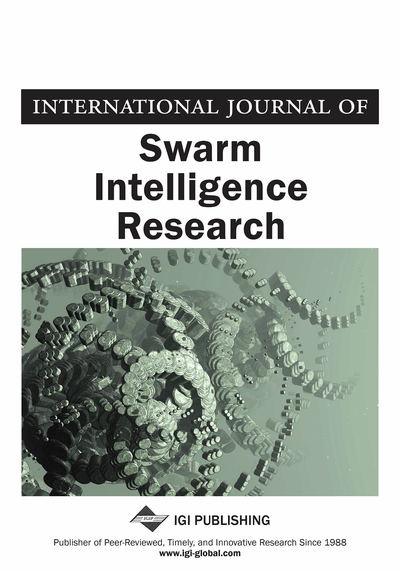# Structural Identification Based on Transient Power Flows using Particle Swarm Optimization

Cibu K. Varghese (Department of Mechanical Engineering, Mar Athanasius College of Engineering, Kothamangalam, India) and K. Shankar (Department of Mechanical Engineering, Indian Institute of Technology Madras, Chennai, Tamilnadu, India)
DOI: 10.4018/jsir.2012100103

## Abstract

This paper presents a transient power flow balance formulation for identifying structural parameters from time domain responses. It is implemented at the substructure level where the concept is to balance the instantaneous powers by equating the input power to the dissipated power and the time rate of change of kinetic and strain energies. This imbalance is reduced to zero to identify the structural parameters. For better results the power balance method is combined with the conventional acceleration matching method where the objective is to minimize the deviation between measured and estimated accelerations - no additional sensors are required to incorporate the extra power flow balance criteria. Numerical simulations are performed for substructures taken from a lumped mass system, a planar truss and a cantilever beam. In numerical simulations, noise free and 3% noise contaminated response measurements are considered. The Particle Swarm approach is used as the optimization method with weighted aggregation multi-objective optimization (MO). The results demonstrate that the proposed combined method is more accurate in identifying the structural parameters of a system compared to previous methods.
Article Preview
Top

## Introduction

System identification is the process of determining the structural parameters of a system based on given input and output (I/O) information of the system. System identification (SI) comes under the category of inverse problems where the values of physical model parameters are obtained from observed data. Input and output data of dynamically excited structures are measured and numerically processed to identify the structural parameters. System identification from measured input/output data has gained great importance due to the availability of powerful identification algorithms. In this study the parameters of interest are instantaneous power flow balance and conventional acceleration matching. Whereas acceleration is a discrete property at one point and a vector, power flow balance is a gross property of the structure which obeys the law of conservation and the various power flow components are scalar. Thus these represent two different approaches and combining them could increase the uniqueness of the inverse problem solution.

Structural power flow analysis has its origin in SEA (Statistical Energy Analysis) pioneered by Lyon (1975), which concerns the ensemble behavior of a group of similar systems when subjected to modally in coherent excitation. SEA originated from Lyon and Maidanik (1962) who calculated power flow between two independently and randomly excited harmonic oscillators assuming weak linear coupling and showed that the energy flow is proportional to the difference in their average modal energies. SEA is an energy response model, i.e., the fundamental variable is the time averaged energy of vibration. Fahy (1994) pointed out in the context of a paper on SEA some of the advantages of studying power flows- various components of power flow can be summed up and power flows between various members obey the law of conservation of energy. The study of energy flows has further advantages in that energy is a scalar quantity and it is thus possible to simply sum up the energy contributions of different wave types, leading to simpler relationships. It is also obvious that energy levels are directly linked to sound emissions and stresses.

Later attention was focused on deterministic power flows in structures for passive damping applications. Singh and Kim (2003) defined time averaged vibrational power input to a structure as the product of harmonic excitation and resultant velocity at the driving point. George and Shankar (2006) used the concept of power flows through the structure to determine the time averaged energy levels of each member in the structure fitted with tuned vibration absorbers. The method used in the aforementioned study calculates power flows using the time averaged product of force and velocity at the input and coupling points of a general structure made of axially vibrating rods. Nirmal and Biswas (2005) described three types of approach that have been reported for vibration power flow measurement, contact, non-contact and numerical methods. Xiong, Xing, and Price (2005) proposed a power flow mathematical model for describing the time averaged power flow in terms of systems damping and velocity response, to reveal the vibrational energy dissipation mechanisms of a dynamic system.

Langley (1997) established that the power dissipated by a linear oscillator subjected to a wide band excitation is independent of the oscillator loss factor. In the aforementioned study power balance equations are derived and equated the power input to the system, power dissipated by damping and the rate of increase of the system energy (kinetic plus potential). The instantaneous power into the system is equal to the instantaneous power dissipated and or absorbed by the damper plus the time rate of change of the sum of the kinetic and strain energies (Stephen, 2006). A system when subjected to a disturbance gains kinetic energy due to the mismatch between power input and output (Al-Taee, Al-Azzawi, Al-Taee, & Al-Jumaily, 2001).The current paper uses transient power flow balance and the formulation is significantly different from time averaged power flow concept (Varghese & Shankar, 2011). Time averaged power flow concept is applicable only for periodic and stationary random excitation in steady state conditions, whereas transient method is applicable to any excitation.

## Complete Article List

Search this Journal:
Reset
Open Access Articles: Forthcoming
Volume 11: 4 Issues (2020): 2 Released, 2 Forthcoming
Volume 10: 4 Issues (2019)
Volume 9: 4 Issues (2018)
Volume 8: 4 Issues (2017)
Volume 7: 4 Issues (2016)
Volume 6: 4 Issues (2015)
Volume 5: 4 Issues (2014)
Volume 4: 4 Issues (2013)
Volume 3: 4 Issues (2012)
Volume 2: 4 Issues (2011)
Volume 1: 4 Issues (2010)
View Complete Journal Contents Listing